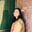Trusted answers to developer questions
Trusted Answers to Developer Questions

Related Tags

cryptography
symmetric
key exchange
diffie-hellman

# What is the Diffie-Hellman key exchange protocol?Umme Ammara

Grokking Modern System Design Interview for Engineers & Managers

Ace your System Design Interview and take your career to the next level. Learn to handle the design of applications like Netflix, Quora, Facebook, Uber, and many more in a 45-min interview. Learn the RESHADED framework for architecting web-scale applications by determining requirements, constraints, and assumptions before diving into a step-by-step design process.

Cryptography provides secure end-to-end communication by employing encryption and decryption. The encryption algorithm converts the input (plaintext) into an encrypted output (ciphertext) using a key. The key must remain secure and unknown to the attacker for the system to stay secure. The two types of cryptosystems are:

• Symmetric Cryptography: There is one shared key used for both encryption and decryption.

• Asymmetric Cryptography: There are two keys (private key and public key), one for encryption and the other for decryption.

A key exchange protocol is used for symmetric cryptosystems to establish the shared key by communicating it over an insecure channel, requiring no previous connections.

For example, if A and B want to exchange encrypted messages, A and B will not need to meet in person secretly to share the key. Instead, they can use an insecure channel effectively and share the key using the Diffie-Hellman key exchange protocol (DH protocol).

## Diffie-Hellman protocol

Based on modular exponentiation, the DH protocol is named after its inventors Whitfield Diffie and Martin Hellman. The steps involved in exchanging the key using this protocol are as follows:

Suppose person A wants to communicate with person B over an insecure channel. The first requirement would be to pick the following two public parameters:

• p: large prime number
• g: a generator number in the range 0 < g < p-1.
1. Person A will then pick a secret number x in the range 0 < x < p-2 and calculate X = $g^{{x}}$ mod p.

2. A will send X over to B.

3. Person B will pick a secret number y in the range 0 < y < p-2 and calculate Y = $g^{{y}}$ mod p.

4. B will send Y over to A.

5. This way, A and B will successfully exchange the necessary parameters to calculate the shared key. A calculates the key as K1 = $Y^{{x}}$ mod p. B calculates the key as K2 = $X^{{y}}$ mod p. A and B have calculated the same key K = $g^{{xy}}$ mod p since K1 = K2.

Note that steps 1 and 3 and steps 2 and 4 can be performed parallel, with no fixed order.

The illustration below depicts the above-mentioned steps, as follows:

DH Protocol

The security of the DH Protocol relies on the fact that the functions X and Y are one-way. It is computationally infeasible to calculate the corresponding keys for an attacker who knows the leaked values of X and Y. Although the DH Protocol is safe against passive attackers, it is vulnerable against active attackers who can modify messages and interfere with the shared key.

RELATED TAGS

cryptography
symmetric
key exchange
diffie-hellman

CONTRIBUTORUmme Ammara